## RD Sharma Class 8 Solutions Chapter 7 Factorizations Ex 7.8

These Solutions are part of RD Sharma Class 8 Solutions. Here we have given RD Sharma Class 8 Solutions Chapter 7 Factorizations Ex 7.8

Other Exercises

Resolve each of the following quadratic trinomials into factors :
Question 1.
2x2 + 5x + 3
Solution: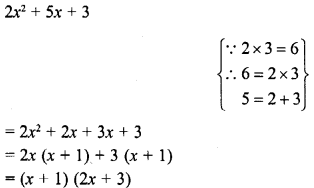Question 2.
2x2– 3x – 2
Solution: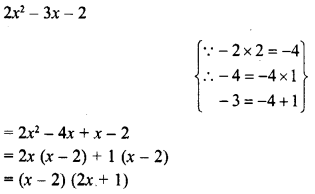Question 3.
3x2 + 10x + 3
Solution: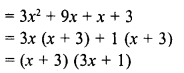Question 4.
7x – 6 – 2x2
Solution:Question 5.
7x2 – 19x – 6
Solution: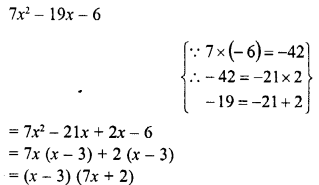Question 6.
28-31x -5x2
Solution: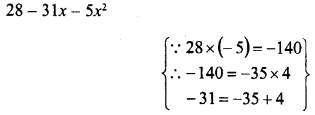Question 7.
3 + 23y – 8y2
Solution: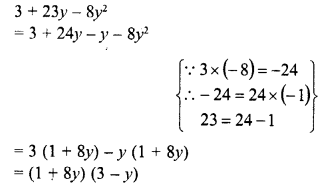Question 8.
11x2 – 54x + 63
Solution: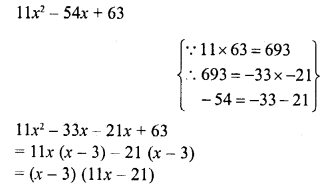Question 9.
7x-6x2 + 20
Solution:Question 10.
3x2 + 22x + 35
Solution: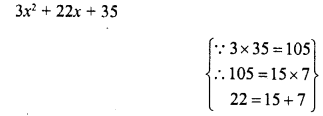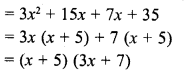Question 11.
12x2 – 17xy + 6y2
Solution: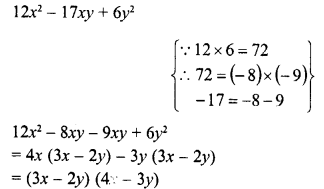Question 12.
6x2 – 5xy – 6y2
Solution: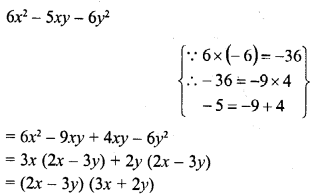Question 13.
6x2 + 13xy + 2y2
Solution:Question 14.
14x2 + 11xy – 15y2
Solution:Question 15.
6a2 + 17ab – 3b2
Solution: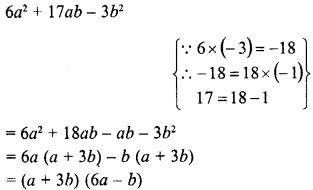Question 16.
36a2 + 12abc – 15b2c2
Solution: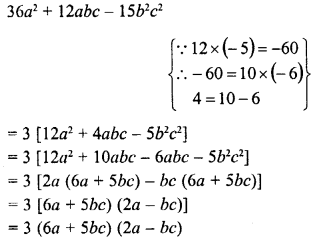Question 17.
15x2 – 16xyz – 15y2z2
Solution: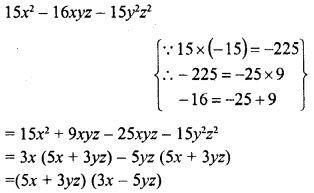Question 18.
(x – 2y)2 -5 (x- 2y) + 6
Solution:Question 19.
(2a – b)2 + 2 (2a – b) – 8
Solution:Hope given RD Sharma Class 8 Solutions Chapter 7 Factorizations Ex 7.8 are helpful to complete your math homework.

If you have any doubts, please comment below. Learn Insta try to provide online math tutoring for you.